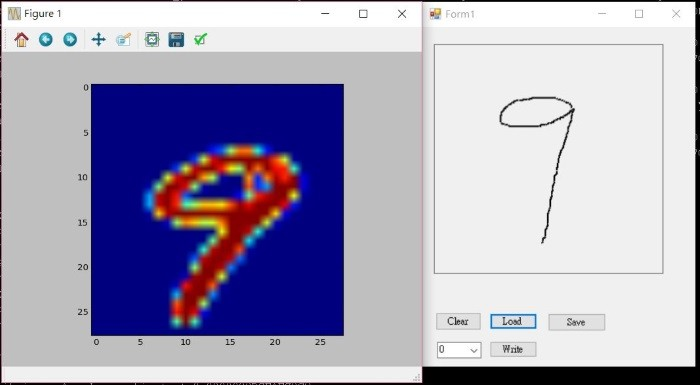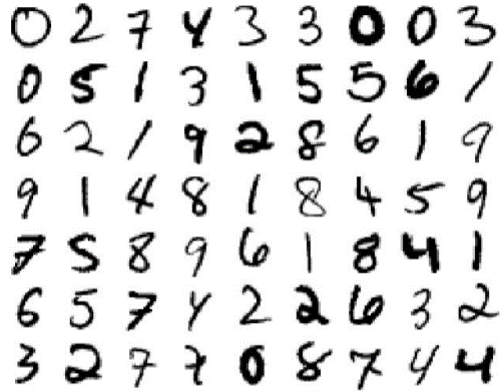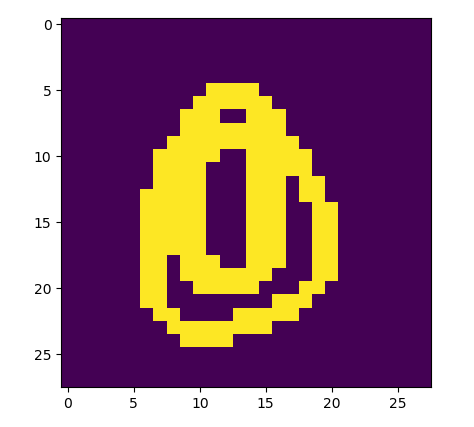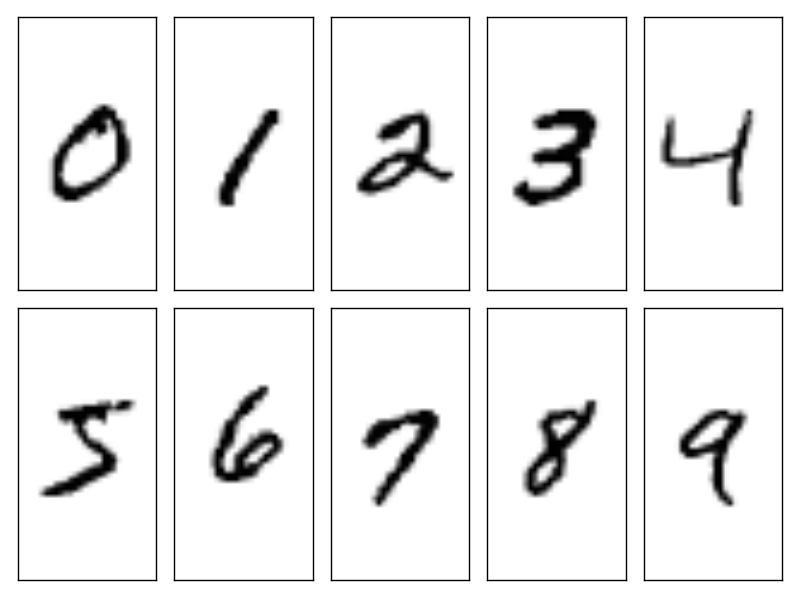#2018 iT 邦幫忙鐵人賽DAY 4
7
AI & Machine Learning

## 前言

1. 模型存檔
2. Keras 組態
3. 資料集(Datasets)
4. Keras事先訓練好的應用程式(Applications)

## 模型存檔(Persistence)

1. 模型結構存檔：以下程式將結構存到 model.config 檔案，檔案為JSON或YAML格式。
``````from keras.models import model_from_json
json_string = model.to_json() with open("model.config", "w") as text_file:
text_file.write(json_string)
``````
1. 權重(W)存檔：以下程式將權重存到 model.weight 檔案。
``````model.save_weights("model.weight")
``````
1. 同時儲存結構與權重，檔案的類別為HDF5。
``````from keras.models import load_model

model.save('model.h5')  # creates a HDF5 file 'model.h5'
``````

## 模型載入

``````import numpy as np
from keras.models import Sequential
from keras.models import model_from_json
with open("model.config", "r") as text_file:
json_string = text_file.read()
model = Sequential()
model = model_from_json(json_string)
model.load_weights("model.weight", by_name=False)
``````

``````from keras.models import load_model

# 刪除既有模型變數
del model

# 載入模型
model = load_model('my_model.h5')
``````

## Keras 組態

1. Keras 組態檔名稱為 keras.json，會儲存在使用者資料夾下的『.keras』子目錄。
2. 如果你下載Keras事先訓練好的應用程式(Applications)，它就會放在使用者資料夾下的『.keras\models』子目錄。
3. 如果你下載Keras的資料集(Datasets)，例如，之前程式下載 MNIST 阿拉伯數字資料集，它就會放在使用者資料夾下的『.keras\datasets』子目錄。不用 (X_train, y_train), (X_test, y_test) = mnist.load_data()，要直接開啟檔案，程式碼如下：
``````f = np.load(get_file("mnist.npz", origin="~/.keras"))
x_train = f['x_train']
y_train = f['y_train']
x_test = f['x_test']
y_test = f['y_test']
f.close()
``````

``````f = np.load(get_file("mnist.npz", origin="https://s3.amazonaws.com/img-datasets/mnist.npz"))
``````

## Keras事先訓練好的應用程式(pre-trained Applications)

Keras提供幾個事先訓練好的經典應用程式，不必重新訓練，可直接套用，請參考官方文件，使用方法如下：

``````from keras.applications.vgg16 import VGG16
from keras.applications.vgg16 import preprocess_input
import numpy as np

model = VGG16(weights='imagenet', include_top=False)
``````

## 資料集(Datasets)

Keras提供幾個現成的資料集，可作為訓練/測試資料，，請參考官方文件，包括手寫數字、分類圖片、影評、新聞、... 等。也可以自其他網站下載，例如，你覺得辨識0~9不過癮，也想辨識 A~Z, a~z，可至這裡下載。

## 實驗

1. 執行 Draw.exe，書寫 0~9，並存成 0.csv, 1.csv ..., 9.csv。
2. 在DOS下，執行 python 0_1.py，假設此程式與*.csv放在同目錄。
3. 可以看到10個數字的辨識結果，如果都正確，那就恭喜你了。## 結論

1. 觀察原圖，MNIST資料應是請測試者在紙上書寫，再掃描進電腦，因為，原檔如下，有毛邊。
2. 使用Draw.exe書寫與MNIST有差異，造成辨識率不佳。### 7 則留言

0MNIST 的圖片應該是請測試者在紙上寫下來，再經掃描，所以，放大看如下，筆劃的寬度不固定且無鋸齒狀，與Draw.exe 不同，所以，辨識率較差。0

# 實驗 我用C#寫了一個Draw.exe 小程式， Source Code 放在這裡，可以使用滑鼠，書寫數字，並將它存成與MNIST類似的格式(.csv)，再用Python程式載入...

``````for i in range(0, 10):
X2 = np.genfromtxt('./'+str(i)+'.csv', delimiter=',').astype('float32')
X1 = X2.reshape(1,28*28) / 255
predictions = model.predict_classes(X1)
# get prediction result
print(predictions)
``````
0

# 報告老師, 執行會有錯誤 (有將*.csv跟0_1.py放在同一檔案夾)

C:\Users\pp>C:\Users\pp\Desktop\0_1\0_1.py
C:\Users\pp\Anaconda3\lib\site-packages\h5py_init_.py:36: FutureWarning: Conversion of the second argument of issubdtype from `float` to `np.floating` is deprecated. In future, it will be treated as `np.float64 == np.dtype(float).type`.
from ._conv import register_converters as _register_converters
Using TensorFlow backend.
Traceback (most recent call last):
File "C:\Users\pp\Desktop\0_1\0_1.py", line 14, in
X2 = np.genfromtxt('./'+str(i)+'.csv', delimiter=',').astype('float32')
File "C:\Users\pp\Anaconda3\lib\site-packages\numpy\lib\npyio.py", line 1689, in genfromtxt
fhd = iter(np.lib._datasource.open(fname, 'rt', encoding=encoding))
File "C:\Users\pp\Anaconda3\lib\site-packages\numpy\lib_datasource.py", line 260, in open
return ds.open(path, mode, encoding=encoding, newline=newline)
File "C:\Users\pp\Anaconda3\lib\site-packages\numpy\lib_datasource.py", line 616, in open
raise IOError("%s not found." % path)
OSError: ./0.csv not found.

0












00